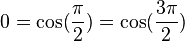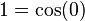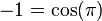As part of the Unified Community Platform project, this wiki and 38 others will be migrated to the new platform in the next few weeks. Minimal changes are expected as part of this migration. Read more here.

# Operator: Cosine

Operator: Cosine

ModPsi
TypeSelector
GroupTrigonometry
Return typeNumber
Parameters
TargetNumber
Technical details
Registry nameoperatorCos

Operator: Cosine is a Number Spell Piece added by Psi. It will return the cosine of a number.

•$0 = \cos({\frac{\pi}{2}}) = \cos({\frac{3\pi}{2}})$
•$1 = \cos(0)$
•$-1 = \cos(\pi)$